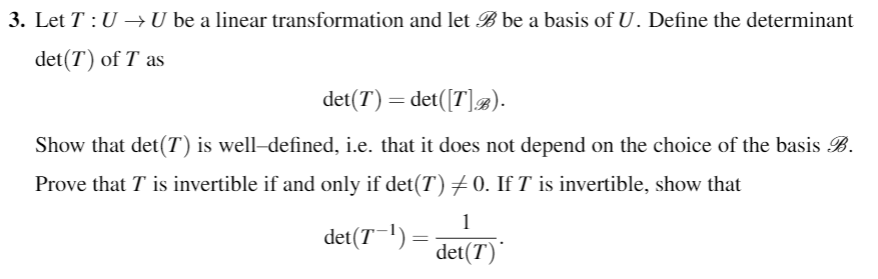# 3. Let T: U - U be a linear transformation and let Bbe a basis of U. Define the determinantdet(T) of T asdet(T) = det(Fla).i.e. that it does not depend on the choice of theShow that det(T) is well-defined,Prove that T is invertible if and only if det(T)メO. If T is invertible, show thatbasis Bdet(71) =det(T)

Question
42 viewshelp_outlineImage Transcriptionclose3. Let T: U - U be a linear transformation and let Bbe a basis of U. Define the determinant det(T) of T as det(T) = det(Fla). i.e. that it does not depend on the choice of the Show that det(T) is well-defined, Prove that T is invertible if and only if det(T)メO. If T is invertible, show that basis B det(71) = det(T) fullscreen
check_circle

Step 1

To establish some basic facts about the determinant of a linear transformation T from U to U (U a vector space)

Step 2

A linear transformation T;U->U is a map satisfying the properties shown.

Step 3

Let B be a basis of U. Then T is represented as a squ...

### Want to see the full answer?

See Solution

#### Want to see this answer and more?

Solutions are written by subject experts who are available 24/7. Questions are typically answered within 1 hour.*

See Solution
*Response times may vary by subject and question.
Tagged in

### Math